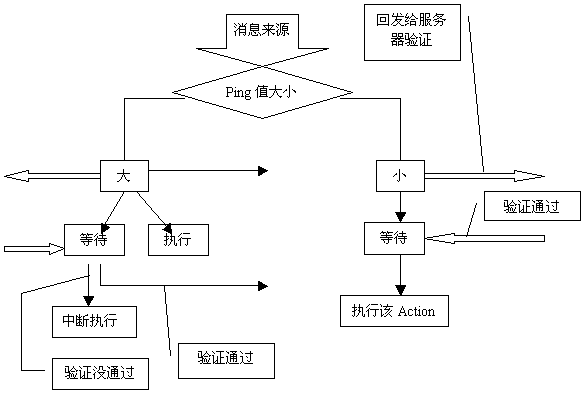# (转) 小谈网络游戏同步T = Dest( TargetB – OriginB ) / Speed

_TargetB = NewPDU.Speed * T

_S = Dest( _TargetB – OriginB ) / T

Struct pos {

Coordinate X;

Coordinate Y;

}

Pos1 = OriginB

Pos2 = OriginB + V

Pos3 = _TargetB – V

Pos4 = _TargetB

x = At^3 + Bt^2 + Ct + D

y = Et^3 + Ft^2 + Gt + H

（其中时间t的取值范围为0-1，在Pos1的时候为0，在Pos4的时候为1）

x(0-3)代表Pos1-Pos4中x的值，y(0-3)代表Pos1-Pos4中y的值

A = x3 – 3 x2 +3 x1 – x0

B = 3 x2 – 6 x1 + 3 * x0

C = 3 x1 – 3 x0

D = x0

E = y3 – 3 y2 +3 y1 – y0

F = 3 y2 – 6 y1 + 3 * y0

G = 3 y1 – 3 y0

H = y0

Pos3 = NewPackage.X + NewPackage.Y t + 1/2 NewPackage.a * t^2

Pos4 = Pos3 – (NewPackage.V + NewPackage.a * t)

1. 本文目前尚无任何评论.
1. 本文目前尚无任何 trackbacks 和 pingbacks.